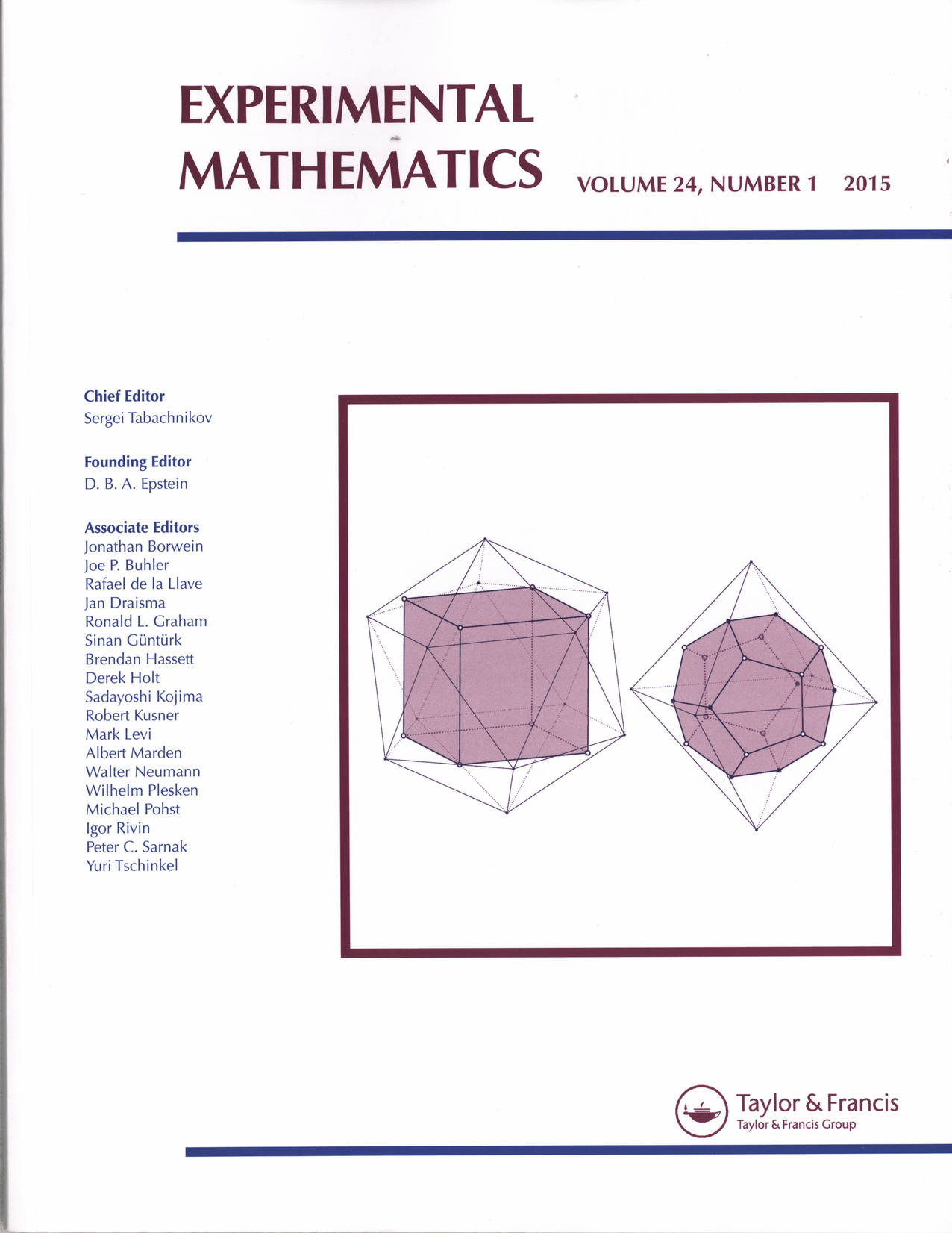## Computing Maximal Copies of Polyhedra Contained in a Polyhedron

 Author: Moritz Firsching Journal: Experimental Mathematics, 24198 -- 105, 2015. Full text: arXiv • DOI • PDFKepler (1619) and Croft (1980) considered the problem of finding the largest homothetic copies of one regular polyhedron contained in another regular polyhedron. For arbitrary pairs of polyhedra, we propose to model this as a quadratically constrained optimization problem. These problems can then be solved numerically; in case the optimal solutions are algebraic, exact optima can be recovered by solving systems of equations to very high precision and then using integer relation algorithms. Croft solved the special cases concerning maximal inclusions of Platonic solids for 14 out of 20 pairs. For the six remaining cases, we give numerical solutions and conjecture exact algebraic solutions.

@Article{ FirschingComputing2015,
author = "Moritz Firsching",
title = "Computing Maximal Copies of Polyhedra Contained in a Polyhedron",
year = "2015",
journal = "Experimental Mathematics",
volume = "24",
number = "1",
pages = "98 -- 105",
doi = "10.1080/10586458.2014.956374"
}